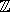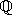CGAL::Homogeneous<RingNumberType>

#include <CGAL/Homogeneous_d.h>

Definition

A model for a Kernel_d using homogeneous coordinates to represent the geometric objects. In order for Homogeneous<RingNumberType> to model Euclidean geometry in Ed, for some mathematical ring E (e.g., the integersor the rationals), the template parameter RT must model the mathematical ring E. That is, the ring operations on this number type must compute the mathematically correct results. If the number type provided as a model for RingNumberType is only an approximation of a ring (such as the built-in type double), then the geometry provided by the kernel is only an approximation of Euclidean geometry.

Kernel_d